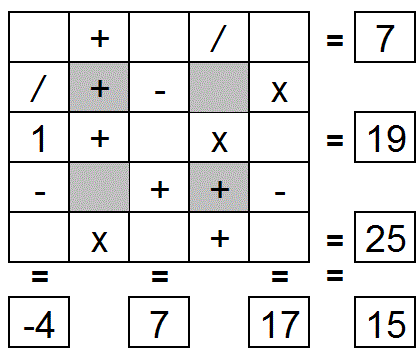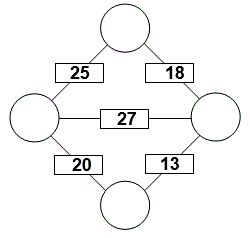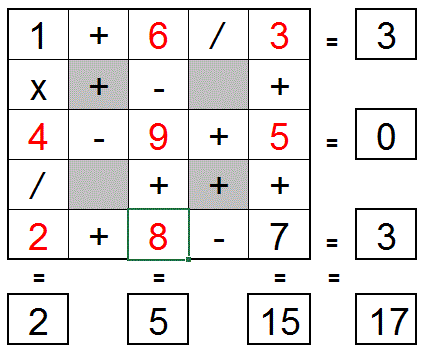Select Page

Each month, a new set of puzzles will be posted.  Come back next month for the solutions and a new set of puzzles, or subscribe to have them sent directly to you.

## ROMAN Arithmetic puzzles

Solve the following arithmetic expressions and state their results in Roman Numerals.

1. IV + XCIX =
2. LIV – XLIV + CLIV =
3. MV – CCCXC – DLII =
4. LXXV + CXIX + DCCXVI =
5. XI + XXII + XXXIII + XLIV – LV =
6. MDCCLXV – MCXX + DXLIV – CDXCVI =
• Letters representing numbers in the Roman numerical system: I = 1, V = 5, X = 10, L = 50, C = 100, D = 500, M = 1,000.
• In this system, a letter placed after another of greater value adds (thus XVI is 16) whereas a letter placed before another of greater value subtracts (thus XC is 90).
• Example:  2018 = MMXVIII

## EQUATE+1 puzzle

Each row, column & diagonal is a mathematical expression or equation and you use the numbers 1 to 9 to complete the equations.   Each number can be used only once. ‘One’ number has been provided to get you started. Find the remaining eight numbers that satisfies all the resulting equations. Note – multiplication (x) & division (/) are performed before addition (+) and subtraction (-).## Feedback

There are more than one way of doing these puzzles and may well be more than one answer.  Please let me and others know what alternatives you find by commenting below.  We also welcome general comments on the subject and any feedback you'd like to give.

If you have a question that needs a response from me or you would like to contact me privately, please use the contact form.

## Get more puzzles!

If you've enjoyed doing the puzzles, consider ordering the book; 150+ of the best puzzles in a handy pocket sized format. Click here for full details.

## MIND-Xpander puzzles

1. In a class of 78 students, 41 are taking French and 22 are taking German. Of the students taking French and German, 9 are taking both courses. How many students are not enrolled in either course?

Solution:

78 = (41 – 9) + (22 – 9) + 9 + (neither)

(neither) = 78 – 32 – 13 – 9 = 24 students

2. You cash your pay-check and save half of it. With the rest, you pay a debt of \$20 and buy something for \$5. Then spending 1/5 of the remaining money, you have \$12 left. How much was your pay-check?

Solution:

Let x = total pay check, then x = 1/2x + 20 + 5 + 1/5 (1/2x – 25) + 12

x = 1/2x + 25 + 1/10x – 5 + 12

x – 1/2x – 1/10x = 32

10/10x – 5/10x – 1/10x = 32

4/10x = 32 & therefore, x = \$80

In the Arithmagon, the value in each box is the sum of the values in the circles on each side of it. Determine the value in each circle.## EQUATE+2 Puzzle

Each row, column & diagonal is an equation and you use the numbers 1 to 9 to complete the equations.   Each number can be used only once. ‘Two’ numbers have been provided to get you started. Find the remaining eight numbers that satisfies all the resulting equations. Note: multiplication (x) & division (/) are performed before addition (+) and subtraction (-).